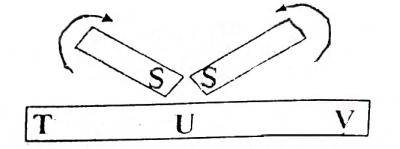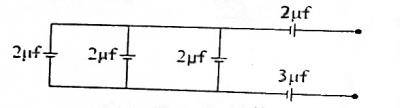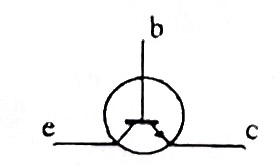## Physics 2018 Past Questions | JAMB

Study the following Physics past questions and answers for JAMBWAEC  NECO and Post-JAMB. Get prepared with official past questions and answers for upcoming examinations.

31. The process whereby a liquid turns spontaneously into vapour is called

• A. regelation
• B. evaporation
• C. boiling
• D. sublimation

32. The differences observed in solids, liquids and gases may be accounted for by

• A. their relative masses
• B. their melting points
• C. the spacing and forces acting between the molecules
• D. the different molecules in each of them

33. Convex mirrors are used as driving mirrors because images formed are

• A. erect, Virtual and diminished
• B. erect, real and diminished
• C. erect, virtual and magnified
• D. inverted, virtual and diminished

34. Musical instruments playing the same note can be distinguished from one another owing to the difference in their

• A. quality
• B. pitch
• C. intensity
• D. loudness

35.In the diagram shown, If the south-poles of two magnets stroke a steel bar, the polarities at T and V will respectively be

• A. north and south
• B. south and south
• C. north and north
• D. south and north

36. In homes, electrical appliances and lamps are connected in parallel because

• A. less current will be used
• B. less voltage will be used
• C. parallel connection does not heat up the wires
• D. series connection uses high voltage

37.  An object moves in a circular path of radius 0.5m with a speed of 1ms$$^{-1}$$. What is its angular velocity?

• A. 8rads$$^{-1}$$
• B. 4rads$$^{-1}$$
• C. 1rads$$^{-1}$$
• D. 2rads$$^{-1}$$

38. What effort will a machine of efficiency 90% apply to a load of 180N if its efforts arm is twice as long as its load arm?

• A. 100N
• B. 90N
• C. 80N
• D. 120N

39.Calculate the effective capacitance of the circuit in the diagram given

• A. 4μf
• B. 3μf
• C. 2μf
• D. 1μf

40.Which of the following diagrams represents correctly an n-p-n transistor?

i.ii.iii.• A. i
• B. ii
• C. iii
Scroll to Top